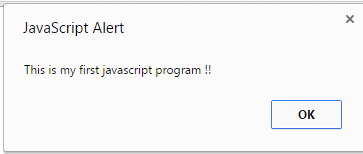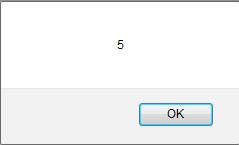# Basic JavaScript Syntax

 SEARCH THIS SITE @import url(http://www.google.com/cse/api/branding.css);Custom Search

Javascript programming syntax is explained through examples. The only way to learn programming is to develop, run and check your javascript programs as you go, through the tutorials presented below. You first need to get started with javascript in order to be able to edit, run and check your javascript practice programs below.

## 1 - Simple Output in Javascrip: alert() method

When developing computer programs, from time to time you want to display outputs to check values of variables for example. The method alert("This is my first javascript program!"); is used in the sample program below to output the string "This is my first javascript program!" as an example copy and run the sample program below and see that the string is displayed as shown in figure 1 below.

<!DOCTYPE html>
<html>
<body>
<script>
alert("This is my first javascript program!");
</script>
</body>
</html>.

## 2 - Semicolon at the end of each Javascript Statement

Semicolons are used at the end of each statement as shown in the sample program below. Use notepad++ (or any other editor) and run it. It should display 5 (because z = x + y = 2 + 3 = 5) as shown in figure 2 below.

<!DOCTYPE html>
<html>
<body>
<script>
var x = 2;
var y = 3;
var z = x + y;
</script>
</body>
</html>

Meaning of each statement
var x = 2; assigns 2 to a variable called x
var y = 3; assigns 3 to a variable called y
z = x + y; calculate x + y and assigns the result to z.

Figure 2 - Display when above sample program is run

## 3 - Comments in Javascript

A line of code starting with // is not executed. Also any code enclosed between /* and */ is not executed. Both // and /* .... */ are used to write comments in javascript programs to make the programs easy to follow and understand. As an example, copy and run the sample program below and see that the statement alert(x); gives an output 2 but if you change it to alert(y); and alert(z); there is no output because var y = 3; and var z = x + y; are treated as comments and not as javascript statements.

<!DOCTYPE html>
<html>
<body>
<script>
var x = 2;
// var y = 3; this will not be executed because of //
// var z = x + y; this will not be executed because of //
/* the next statement alert(x); display the variable
between its brackets (this is a comment and will not be executed) */